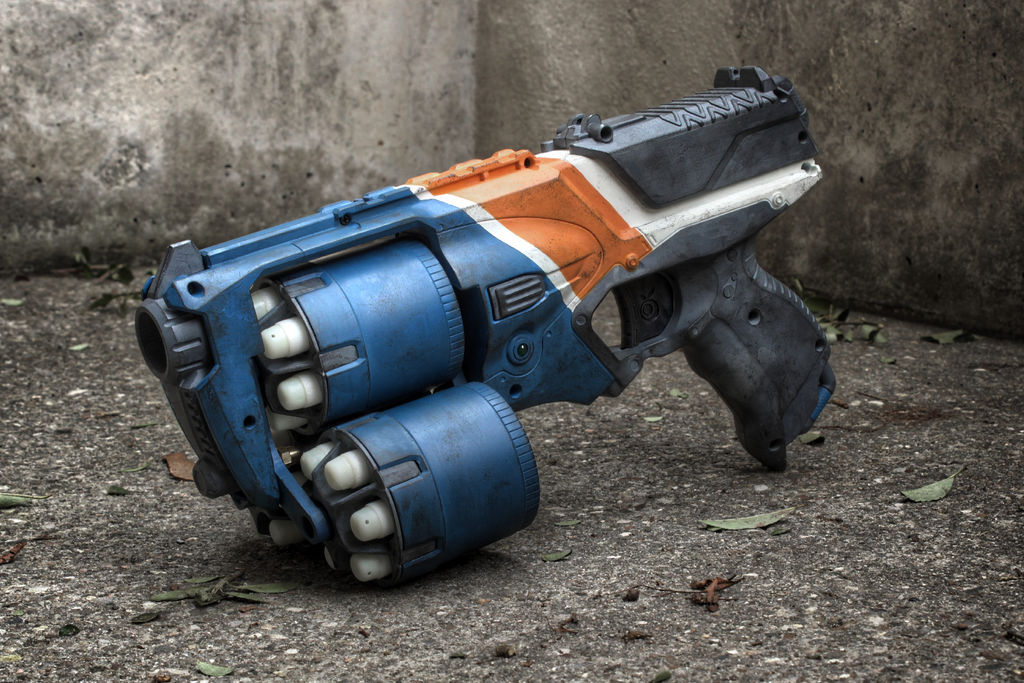Classical Mechanics

# Introduction to Forces: Level 1-2 Challenges

A passenger in a closed train car moving at constant velocity tosses a coin directly upward. Where will the coin fall?

(Ignore effects of air resistance.)One of the most lethal NERF guns is the Strongarm, a 6 shooter revolver known for its rapid fire. The firing mechanism is straightforward, a spring is used to drive air through a cylinder which then forces the dart out of the barrel.

Its popularity is due in part to the ease with which it can be modified to shoot faster and farther. One easy modification is to insert a secondary spring inside the main spring, as shown below:If the strength (spring constant) of the first spring is $k$, and the strength of the second spring is $\frac12 k$, what is the strength of the two springs together?

In the absence of air resistance, a ball of mass $m$ is tossed upward to reach a height of $20 \text { m}$. When it is $10 \text { m}$ in the air (half way up), what is the net force on the ball?An astronaut is accidentally separated from his small spaceship which is accelerating in inter stellar space at a constant rate of $100\text{ m/s}^2$. What is the acceleration of the astronaut the instant after he is outside the spaceship?

Details

• Assume that there are no nearby stars to exert gravitational force on him.You are shrunk to the height of a small pebble and thrown into a blender. Your mass is reduced so that your density is the same as usual. The blades start moving in 60 seconds and you'll die if you stay at the bottom. What would you do?

One valid but counterintuitive answer is to jump out of the blender.

Suppose a person of height 1.80 m and mass 60 kg can jump to the height of 60 cm. If this person is shrunk to one thousandth of his original size, how high is he now able to jump in centimeters?

Assumptions

• The amount of power a human can generate is proportional to the muscle mass $m$.
×